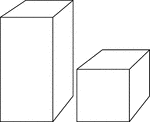### 2 Rectangular Prisms

Illustration of 2 right rectangular prisms. The bases are congruent, but the height of the smaller prism…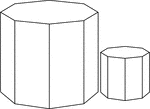### 2 Similar Octagonal Prisms

Illustration of 2 Similar right octagonal prisms. The height and length of the edges of the smaller…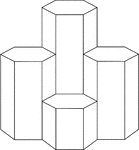### 4 Hexagonal Prisms

A cluster of 4 right hexagonal prisms with congruent bases, but varying heights.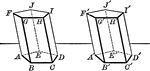### Equal Prisms

Illustration showing two prisms are equal if the three faces which include a trihedral angle of the…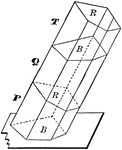### Oblique Prism Truncated Into 3 Sections

Illustration of an oblique prism constructed by the combination of three truncated prisms.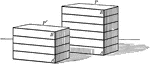### Rectangular prisms

Illustration of 2 parallelopipeds/rectangular prisms.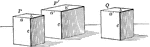### Rectangular prisms

Illustration of 3 parallelopipeds/rectangular prisms.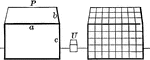### Rectangular Prisms Showing Volume

Illustration of 2 rectangular prism used to show volume.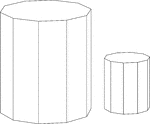### Similar Decagonal Prisms

Illustration of 2 similar right decagonal prisms. Both have regular decagons for bases and rectangular…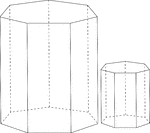### Similar Heptagonal/Septagonal Prisms

Illustration of 2 similar right heptagonal/septagonal prisms. Both have regular heptagons/septagons…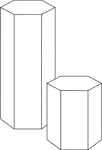### Similar Hexagonal Prisms

Illustration of 2 similar right hexagonal prisms. The height of the prism and length of the side of…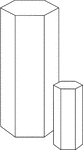### Similar Hexagonal Prisms

Illustration of 2 similar right hexagonal prisms. The height of the prism and length of the side of…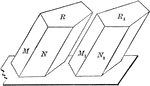### Two Equal Prisms

Diagram used to prove the theorem: "Two prisms are equal when the three faces about a trihedral of one…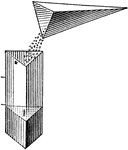### Comparative Volumes Of A Pyramid And Prism

Illustration used to compare the volumes of a pyramid and a prism by emptying sand from the pyramid…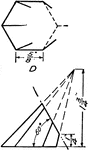### Development Exercise of Hexagonal Pyramid

A sample exercise problem in completing the top part of the hexagonal pyramid and to create a development,…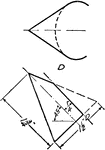### Development Exercise of Oblique Hexagonal Pyramid

A sample exercise problem to complete the top part and create a stretched out image of the oblique hexagonal…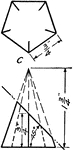### Development Exercise of Pentagonal Pyramid

A pentagonal pyramid problem exercise to complete the top view of the image, and development, or rolled…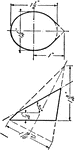### Development Exercise of Pentagonal Pyramid

The problem exercise in completing the top view and creating the development, or rolled out, image of…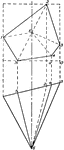### Isometric of Oblique Pentagonal Pyramid

Illustration of the isometric of an oblique pentagonal pyramid.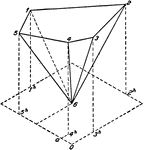### Oblique Pentagonal Pyramid

Illustration of a plan and elevation of an oblique pentagonal pyramid.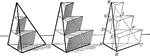### Triangular Pyramid For Volume

Illustration of triangular pyramid used to show that the volume is the limit of the sum of the volumes…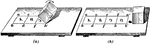### Development Of Rectangular Prism

Illustration of the development of a rectangular prism. "Assume now that the prism is laid on the drawing…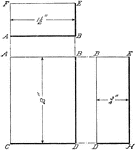### Projection Of Rectangular Prism

Illustration of the projection of a rectangular prism that is represented as if it were standing on…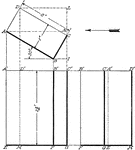### Projection Of Rectangular Prism

Illustration of the projection of a rectangular prism whose broad side makes an angle of 30° with…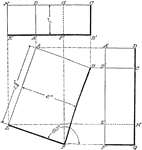### Projection Of Rectangular Prism

Illustration of the projection of a rectangular prism whose base makes an angle of 160° with the…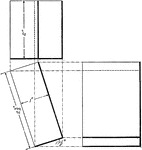### Projection Of Rectangular Prism

Illustration of the projection of a rectangular prism whose base makes an angle of 17.5° with the…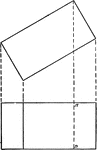### Projections of Rectangular Prism

Projections of a rectangular prism or block.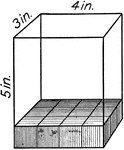### Volume Of A Rectangular Solid

Illustration of a 5 in. by 3 in. by 4 in. rectangular solid with each cube in the solid representing…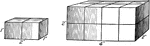### Similar Rectangular Solids

Illustration of a 1 in. by 2 in. by 1 in. rectangular solid that is similar to a 2 in. by 4 in. by 2…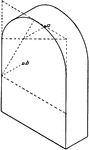### Prism With Semicircular Top

Isometric of a prism with a semicircular top.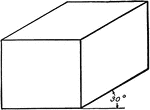### Oblique View Of Rectangular Solid

Illustration of an oblique view of a rectangular solid/prism at 30°.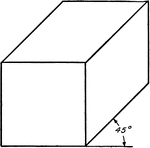### Oblique View Of Rectangular Solid

Illustration of an oblique view of a rectangular solid/prism at 45°.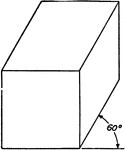### Oblique View Of Rectangular Solid

Illustration of an oblique view of a rectangular solid/prism at 60°.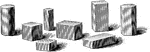### Solids

Various geometric solids, mostly rectangular prisms with a cylinder and a hexagonal prism.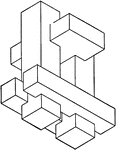### Combination of Geometric Solids

Illustration of model created by combining non regular geometric solids.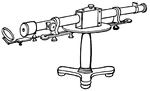### Direct Vision Spectroscope

"...an instrument which gives a spectrum when the source of the light is in a straight line with the…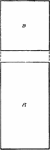### Projection of Square Bar

Projection of a square bar.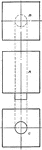### Projection of Square Bar With Cylindrical Portion

Projection of a square bar with a cylindrical portion.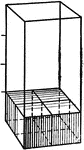### Volume Of Square Prism

Illustration used to show finding the volume of a square prism.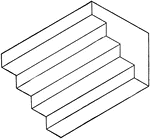### Isometric of Stairs

Illustration of stairs; 3-dimensional view of 4 steps. The staircase can be created by combining/stacking…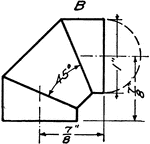### Development Exercise of Cylinder using Three Piece Elbow

The exercise problem of creating a three piece elbow development or rolled out image of the cylinder…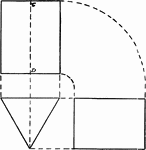### Projections of Triangular Prism

Projections of a triangular prism or block.### Projections of Triangular Prism With Hole

Projections of a triangular prism or block with a square hole.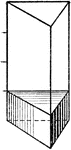### Volume Of Triangular Prism

Illustration used to show finding the volume of a triangular prism.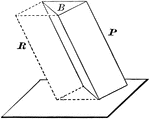### Volume of Triangular Prism

Diagram used to prove the theorem: "The volume of a triangular prism is equal to the product of its…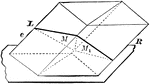### Parallelopiped Divided Into Triangular Prisms

Diagram used to prove the theorem: "The plane passed through two diagonally opposite edges of a parallelopiped…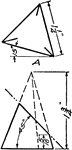### Development Exercise of Triangular Pyramid

Exercise problem to develop, or rolled out, image of the triangular pyramid by creating an equal length…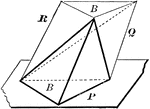### Volume of Triangular Pyramid

Diagram used to prove the theorem: "The volume of a triangular pyramid is equal to one third of a triangular…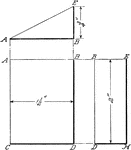### Projection Of Wedge

Illustration of the projection of a wedge of a rectangular prism that is standing on one of its triangular…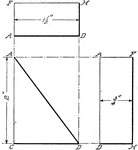### Projection Of Wedge

Illustration of the projection of a wedge of a rectangular prism that is standing on one of its rectangular…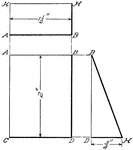### Projection Of Wedge

Illustration of the projection of a wedge of a rectangular prism that is viewed from the side.